## Example Questions

### Example Question #1 : Graphing

A triangle is made up of the following points: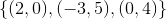What are the points of the inverse triangle?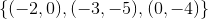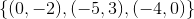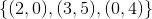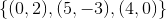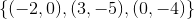Explanation:

The inverse of a function has all the same points as the original function, except the x values and y values are reversed. The same rule applies to polygons such as triangles.

### Example Question #1 : Graphing

Electrical power can be generated by wind, and the magnitude of power will depend on the wind speed. A wind speed of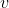(in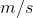) will generate a power of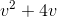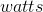. What is the minimum wind speed needed in order to power a device that requires?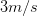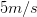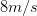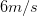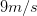Explanation:

The simplest way to solve this problem is to plug all of the answer choices into the provided equation, and see which one results in a power of.

Alternatively, one could set up the equation,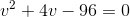and factor, use the quadratic equation, or graph this on a calculator to find the root.

If we were to factor we would look for factors of c that when added together give us the value in b when we are in the form,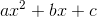.

In our case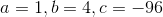. So we need factors of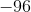that when added together give us.

Thus the following factoring would solve this problem.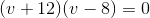Then set each binomial equal to zero and solve for v.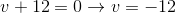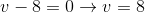Since we can't have a negative power our answer is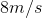.

### Example Question #1 : Graphing

Compared to the graph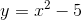, the graph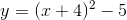has been shifted:units down.units to the right.units to the left.units down.units up.units to the left.
The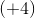inside the argument has the effect of shifting the graphunits to the left. This can be easily seen by graphing both the original and modified functions on a graphing calculator.